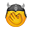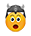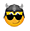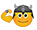## An equation for the wisest

53 Replies
29 July, 2019, 3:00 PM UTC

Lords, the wisest scientists of the Kingdom have been struggling for several days with this equation. Can you solve it?UTC +7:00
1
User
29 July, 2019, 5:27 PM UTC

(5+3)/4=2

2*5-3*3=1

15-2*3=9

2*4*(3*5-3*3)=8*6=48
UTC -2:00
0
User
30 July, 2019, 8:12 PM UTC

I'm bad at mathUTC +0:00
0
User
31 July, 2019, 3:31 AM UTC
48 now where are my Anne candles ☺️
UTC +0:00
0
User
31 July, 2019, 4:24 PM UTC

a+c/b = 2 ; a+3 = 2b; 15 - 2c = 9; a = 5, b  = 4, c=3

2*4(15-9) = 48

? = 48
UTC +0:00
0
User
31 July, 2019, 7:05 PM UTC
48   (2 * 4 * (3*5-3*3) = 48)UTC +1:00
0
User
1 August, 2019, 4:16 AM UTC

15 -  2 shields = 9

Therefore 1 shield = 3

2 masks - 3 shields (9) = 1

1 mask + 1 shield / goblet = 2

5+3/goblet = 2

Therefore goblet = 4

final puzzle

2 goblets (8) x (3 masks (15) - 3 shields (9) )

equals 8 (15-9)

= 8 x 6

=48
UTC +3:00
0
User
1 August, 2019, 10:17 AM UTC

John the Fearless said:

Lords, the wisest scientists of the Kingdom have been struggling for several days with this equation. Can you solve it?1 x Shield   = 3

1 x Goblet  = 4

1.    15 - 6 = 9        [15 - 2 x Shield = 9                            Calculating method: 15-9=6  also 2 Shield are  6  therefore  1 Shield = 3]

2.    10 - 9 = 1        [2 x Mask - 3x Shield = 1                   Calculating method: 1+9=10 also 2 Mask are  10  therefore 1 Mask   = 5]

3.    5 + 3 : 4 = 2    [1xMask + 1xShield : 1xGoblet = 2    Calculating method: 5+3:4=2                                therefore  1 Goblet = 4]

8 (15-9) = 48         [2 x Goblet (3 x Mask - 3x Shield) = 48]

UTC +0:00
0
User
1 August, 2019, 1:47 PM UTC
I'm still trying to figure out what they do with all the taxes they collect for sending rss. I know it's not to improve the roads. My horse broke a shoe hitting a pot hole. Who going to pay for that?
UTC +0:00
3
User
2 August, 2019, 7:09 AM UTC

5+3=8/4= 2

10-9=1

15-6=9

8(15-9)=8×6=48
UTC +0:00
0
User
2 August, 2019, 11:56 AM UTC
48
UTC +0:00
0
User
2 August, 2019, 4:12 PM UTC

(5+3)/4=2

2*5-3*3=1

15-2*3=9

2*4*(3*5-3*3)=8*6=48

knight0704
UTC +1:00
0
User
4 August, 2019, 11:45 AM UTC
? = 48

UTC +0:00
0
User
5 August, 2019, 12:37 PM UTC

48 is the answer for the final equation

H
UTC +4:00
0
User
5 August, 2019, 1:08 PM UTC

shield  = 3

goblet = 4

?= 48

UTC +0:00
0
User
5 August, 2019, 1:34 PM UTC

Goblet x 2 = 4 x 2 = 8

Mask x 3 = 5 x 3 = 15

Shield x 3 = 3 x 3 = 9

8(15-9) = 48 (applying BODMAS)
UTC +8:00
0
User
5 August, 2019, 2:47 PM UTC

1st deduction operation: 15 - 2 shields = 9

By deduction, 15 - 6 = 9

the shield value is 3 either (2x3) = 6

2nd operation, 2 masks - 3 shields = 1

We know the value of the shield which is 3 from the previous operation.

Thus we can write :  2 masks - 3 x 3 =1

3rd operation, 1 mask + 1 shield divided by 1 chalice = 2

We know the value of the mask and shield, respectively 5 and 3.

Can be written (5 + 3)/ 1 chalice = 2

The chalice is therefore = 4, since we can check that 2 x 4 = 8

4th operation, 2 chalices ( 3 masks - 3 shields) = ?

We know the value of

each object, the following operation can be written: 2 x 4 ((3 x 5)-(3 x 3)= 8 (15-9)= 8x6 = 48UTC +2:00
0
User
6 August, 2019, 6:12 AM UTC
UTC +0:00
0
User
7 August, 2019, 2:24 PM UTC

8 ( 15 - 9 )   =48

^     ^

120 - 72 = 48

M&M
UTC -4:00
0
User
9 August, 2019, 11:28 PM UTC
48
UTC +0:00
0
7565114 users registered; 153501 topic; 533650 posts; our newest member:healthcountzami1972